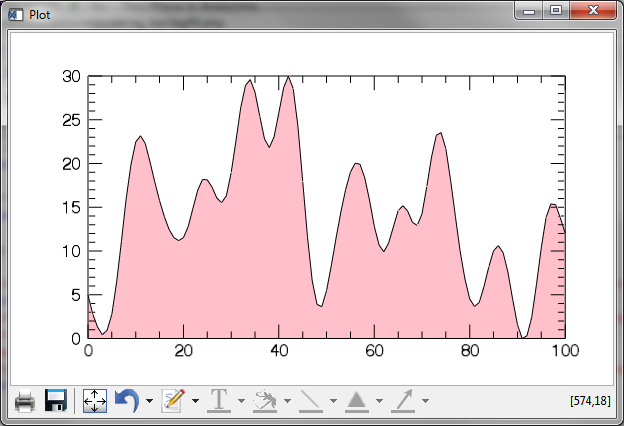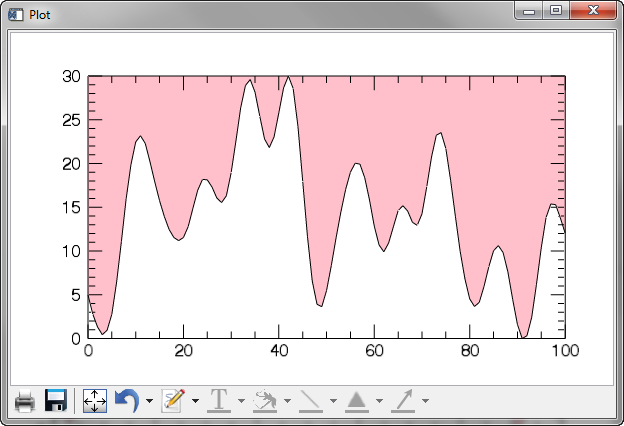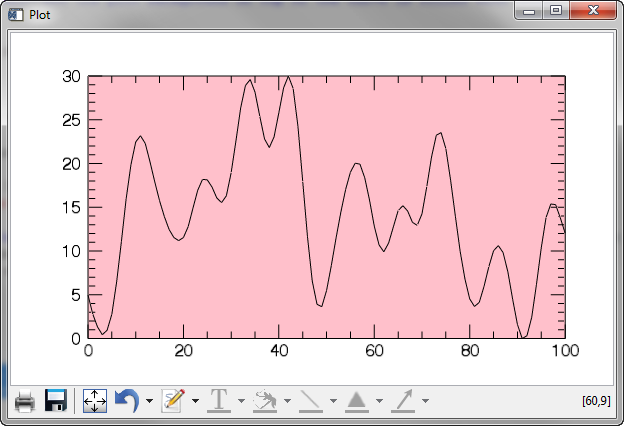Filling the Background of a PlotQUESTION: I am trying to fill the background of a function graphics plot with a specific color. I've read the (exceedingly poor) documenation, but I just can't seem to figure it out. It would seem straightforward, as there are keywords for doing this, it appears: Fill_Background, Fill_Color, etc. I tried the intuitive thing, but only the portion of the background under the curve is filled.

data = cgDemoData(1)
p = Plot(data, Fill_Background=1, Fill_Color='pink')

You see the results in the figure below.Only the plot background under the curve is filled with color.

I don't really understand the purpose of the Fill_Level keyword, but presumably if I set this to the limit of the Y axis, the background should fill the entire plot. It doesn't.

p = Plot(data, Fill_Background=1, Fill_Color='pink', Fill_Level=30)Now the plot background on top of the curve is filled with color.

Is there any way to fill the entire plot background with color?ANSWER: The (non-intuitive) way to do this is to use two plots. The second plot draws a line along the X axis, and then uses the extent of the Y axis of the first plot as input to the Fill_Level keyword. Finally, the first plot is moved in front of the second plot. The code looks like this.

data = cgDemoData(1)
p1 = Plot(data)
p2 = Plot(Overplot=p1, p1.xrange, [0,0], Fill_Background=1, \$
Fill_Color='pink', Fill_Level=30)
p1.Order, /Bring_To_Front

You see the results in the figure below.The entire plot background filled with color.